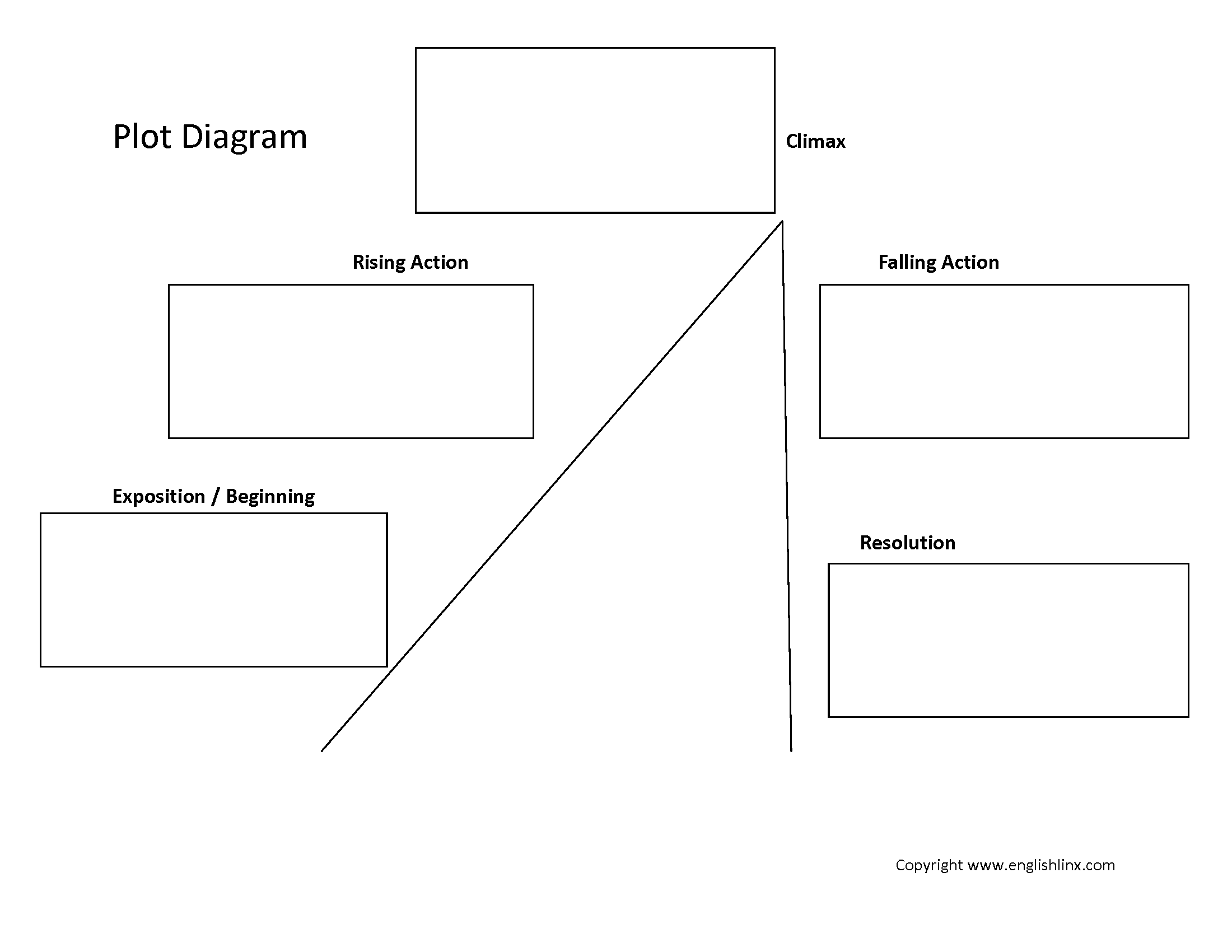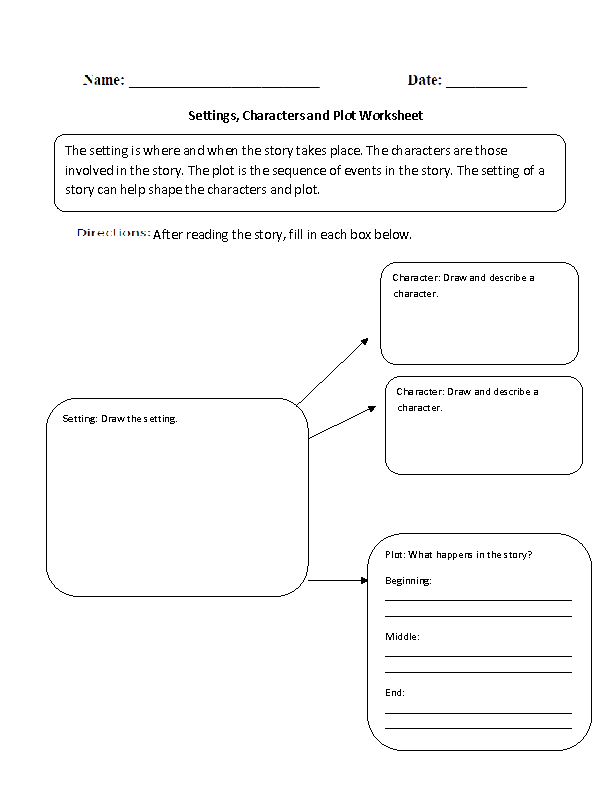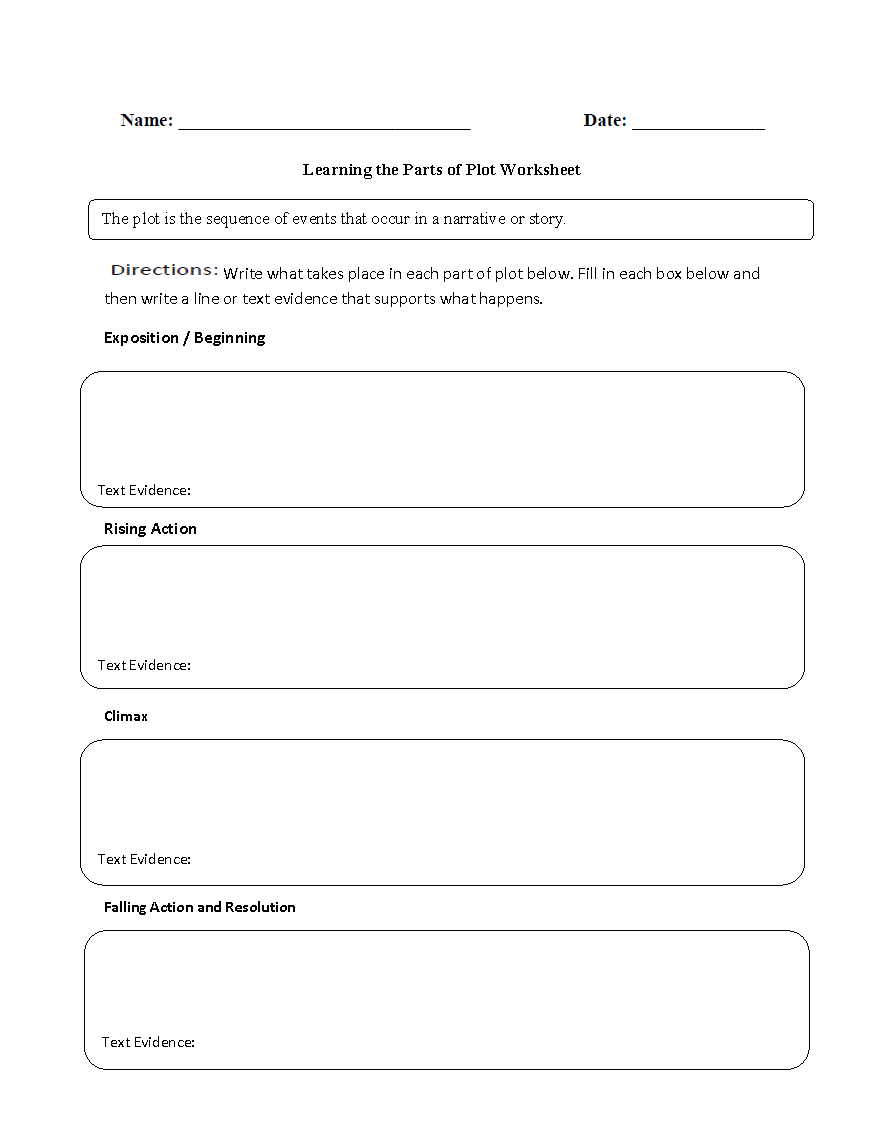# Plot Worksheets

## Plot Worksheets Practice

A plot of a story is the sequence of events that occur. There are five major parts of plot; introduction, rising action, climax, falling action, and resolution. Each part of plot represents a different part of the story. The introduction includes the indroduction of the setting and characters. The rising action usually is about how the drama builds and the first conflicts arise. The climax is the highest point of action in the story. The conclusion is what happens at the end of the story. The plot is a major part of writing and reading narratives or stories in the English language.

Here is a graphic preview for all of the Plot worksheets. Our Plot worksheets are free to download and easy to access in PDF format. Use these Plot worksheets in school or at home. Click here for a description of all Plot Worksheets.

## Plot Worksheets

Click on the image to display our plot worksheets.

##### Plot Diagram 1Plot Worksheet##### Plot Diagram 2Plot Worksheet##### Story MapPlot Worksheet##### Plot,Setting,CharactersPlot Worksheet##### Beginning,Middle and EndPlot Worksheet##### Plot DiagramHalf Sheet Worksheet##### Parts of PlotWorksheet##### Plot Book ReportWorksheet##### Finding the PlotWorksheet##### Learning Parts of PlotWorksheet## Description for all Plot Worksheets

This plot worksheet directs the student to fill out each part of plot. There is a section for each part of plot to fill out. The student must read the text and write in the plot worksheet what happens in the beginning, rising action, climax, falling action and resolution.

This plot worksheet directs the student to fill out each box that represents each part of plot. There is a section for each part of plot to fill out. The student must read the text and write in the plot worksheet what happens in the beginning, rising action, climax, falling action and resolution.

This plot worksheet directs the student to write all about a story they have read. The story map includes questions on setting, characters, conflict, climax, and resolution.

This plot worksheet instructs the student to write about how the setting relates and influences the plot of the story. The student must first draw the setting of the story. Once completed, the student must draw and describe two characters. Lastly, the plot worksheet has the student write what happens in the beginning, middle and end of the narrative.

This plot worksheet directs the student to write in the boxes what happens in the beginning, middle and end of the story or narrative. This plot worksheet gives the student good reading comprehension practice.

This plot worksheet instructs the student to write the different parts of plot on a half sheet. The half sheet is designed to save paper and copies. This plot worksheet can give students good reading comprehension practice by having them fill out each part of plot.

This plot worksheet directs the student to write what happens in each part of plot. The student must write what happens in the beginning of the story. Then they must write what happens in the middle of the story.

This plot worksheet directs the student to write a book report that centers around the plot of the story. The student must first read a book or novel, and then fill out the parts of plot. This plot book report then requires the student to write about the protagonist, setting, a memorable quote, the theme and find one difficult word from the reading and write its meaning.

This plot worksheet teaches the student about the dynamic and fun nature of plot. This plot worksheet instructs the student to write down the events that take place in the story from beginning to end. Once they written down the events, the student then judges each event by how boring or exciting it was.

This plot worksheet has the student write what happens in each part of plot. After reading the given text, the student must write what happens in the exposition, rising action, climax, falling action and resolution. In addition, the student must give text evidence or a line from the reading that supports what they have written.Custom Search